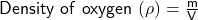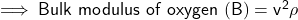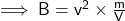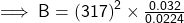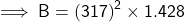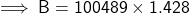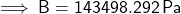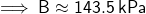6. What is the bulk modulus of oxygen if 32.0 g of oxygen occupies 22.4 L and the speed of sound in the oxygen is 317 m/s?

Question

6. What is the bulk modulus of oxygen if 32.0 g of oxygen occupies 22.4 L and the speed of sound in the oxygen is 317 m/s?

in progress 0
5 months 2021-08-09T15:58:49+00:00 1 Answers 16 views 0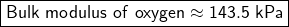Given:

Mass of oxygen (m) = 32.0 g = 0.032 kg

Volume occupied by oxygen (V) = 22.4 L = 0.0224 m³

Speed of sound in oxygen (v) = 317 m/s

To Find:

Bulk modulus of oxygen

Explanation: